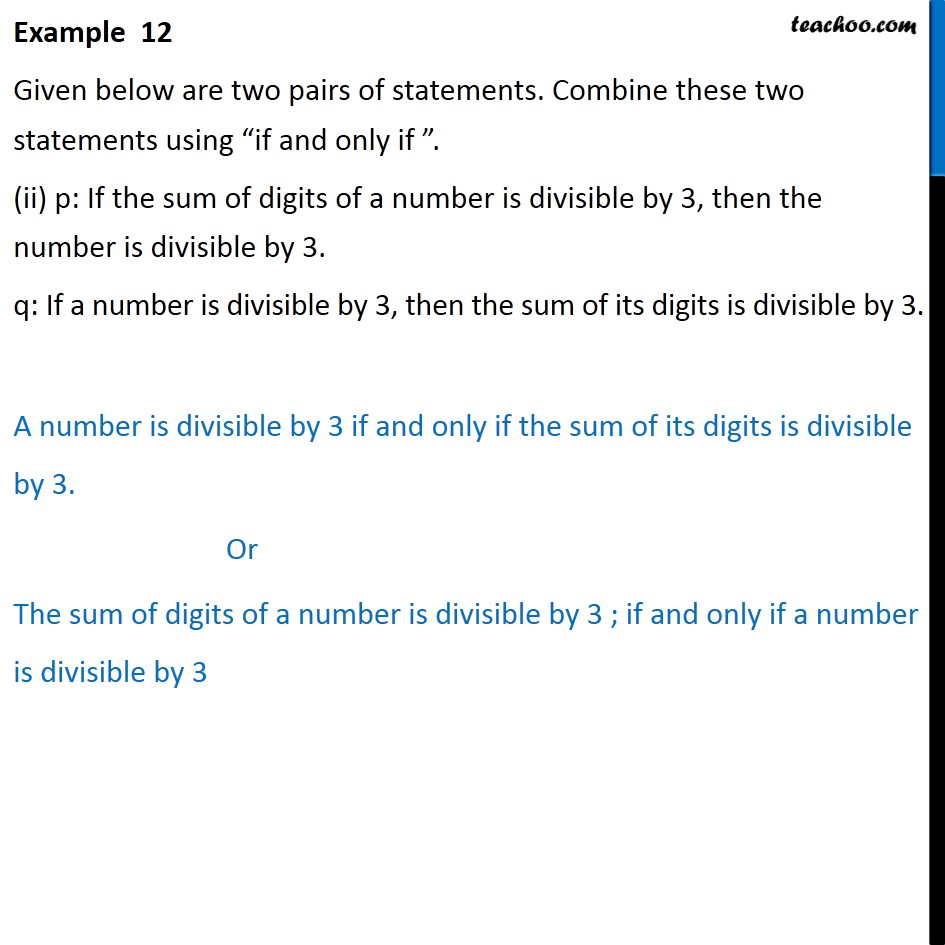Examples

Mathematical Reasoning
Serial order wiseLearn in your speed, with individual attention - Teachoo Maths 1-on-1 Class

### Transcript

Example 12 Given below are two pairs of statements. Combine these two statements using if and only if . (ii) p: If the sum of digits of a number is divisible by 3, then the number is divisible by 3. q: If a number is divisible by 3, then the sum of its digits is divisible by 3. A number is divisible by 3 if and only if the sum of its digits is divisible by 3. Or The sum of digits of a number is divisible by 3 ; if and only if a number is divisible by 3